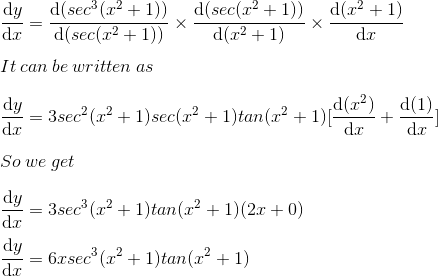# RS Aggarwal Solutions for Class 12 Maths Chapter 10: Differentiation Exercise 10A

Exercise 10A has problems based on derivatives of some functions and important rules of differentiation. The solutions are created by our subject matter experts with the mindset of helping students learn the concepts with ease. Various examples before the exercise wise problems improve problem analysing abilities among students. Given below is the link of RS Aggarwal Solutions Class 12 Maths Chapter 10 Differentiation Exercise 10A PDF, to help students solve problems without any difficulty.

## RS Aggarwal Solutions for Class 12 Maths Chapter 10: Differentiation Exercise 10A Download PDF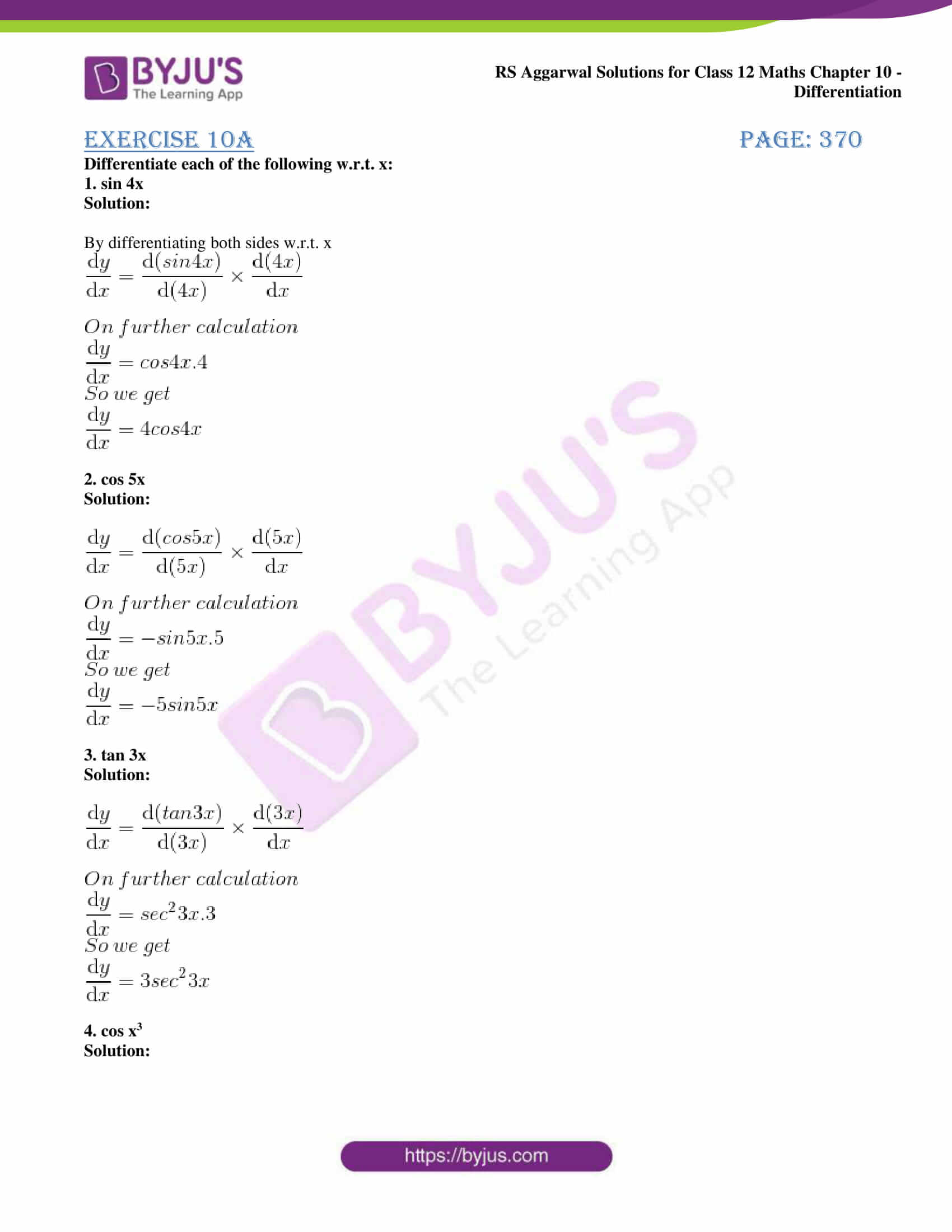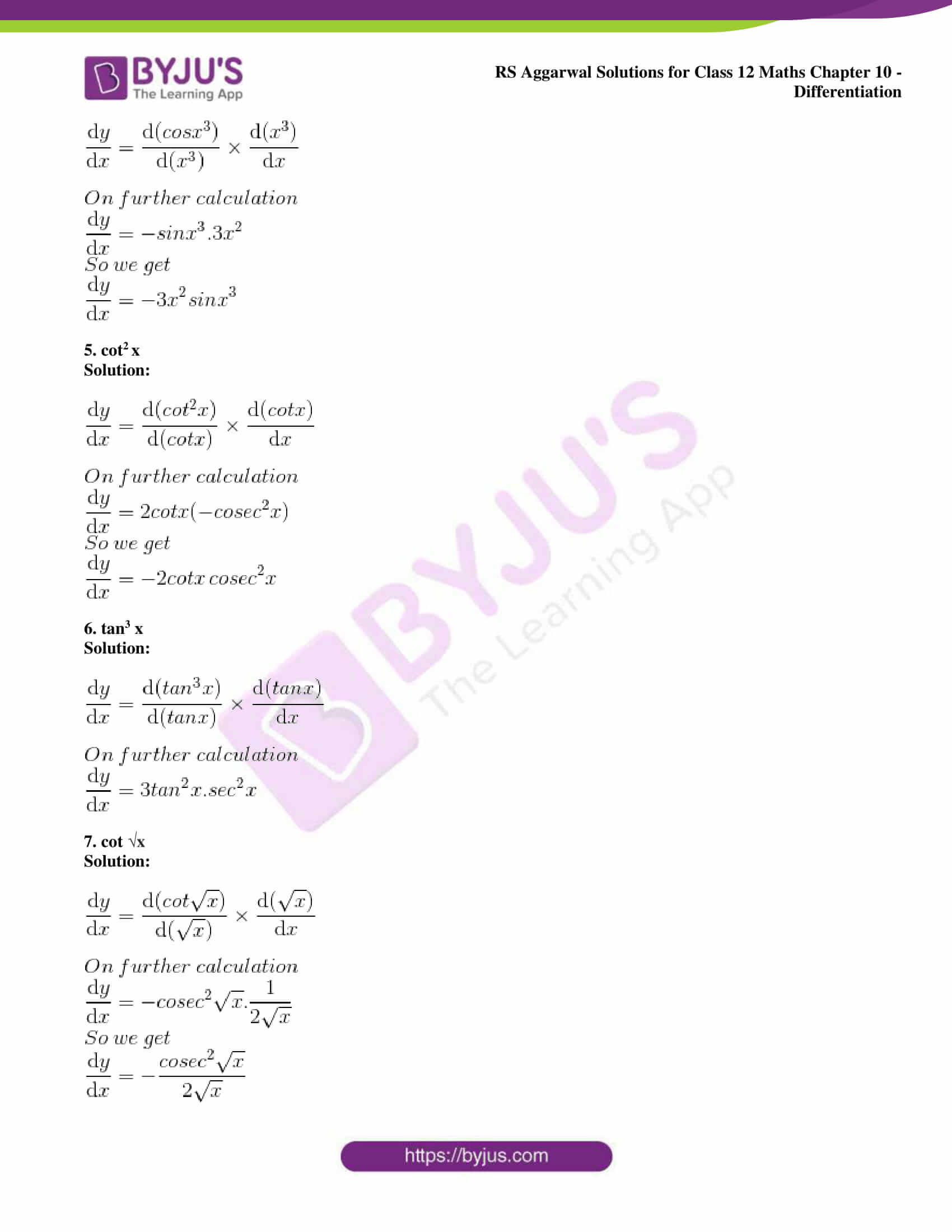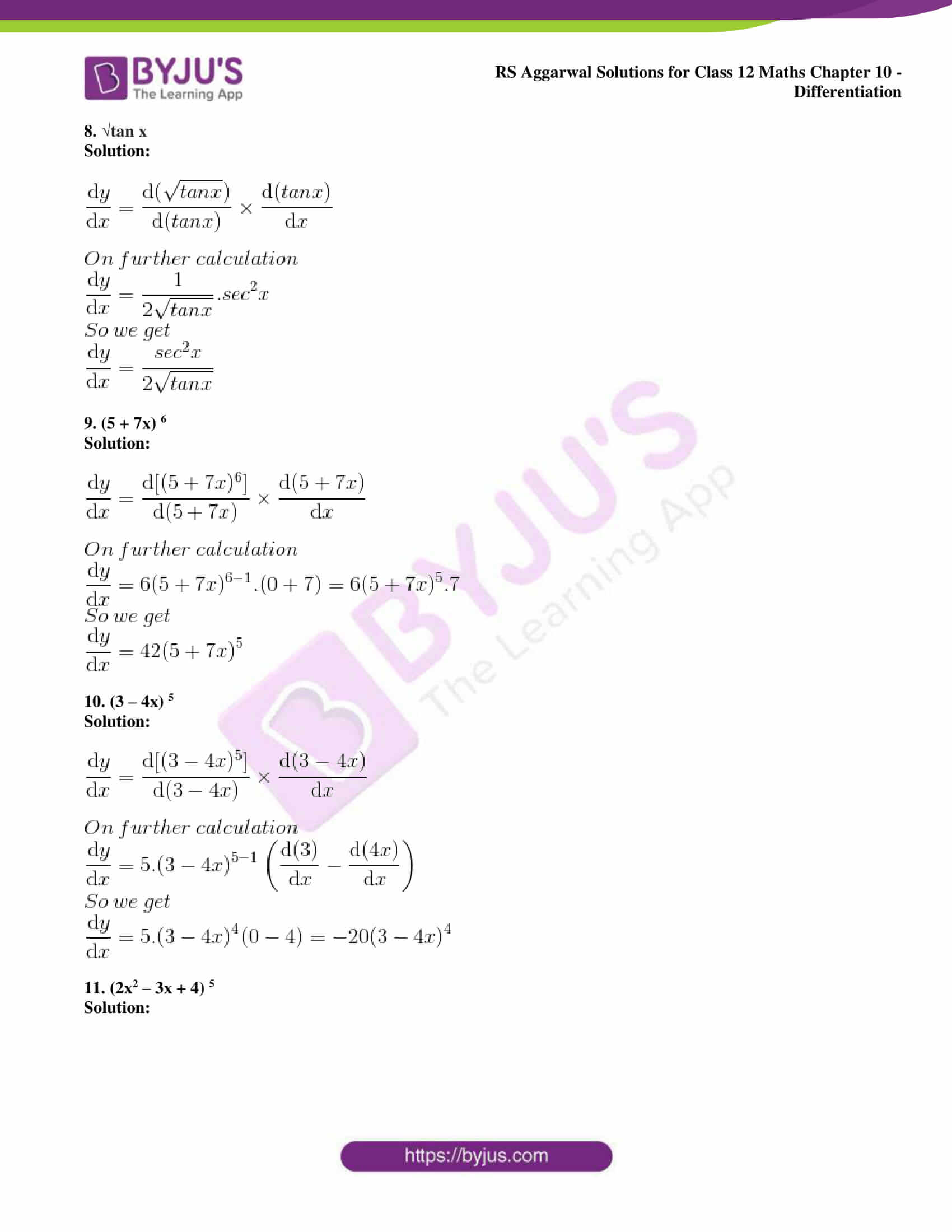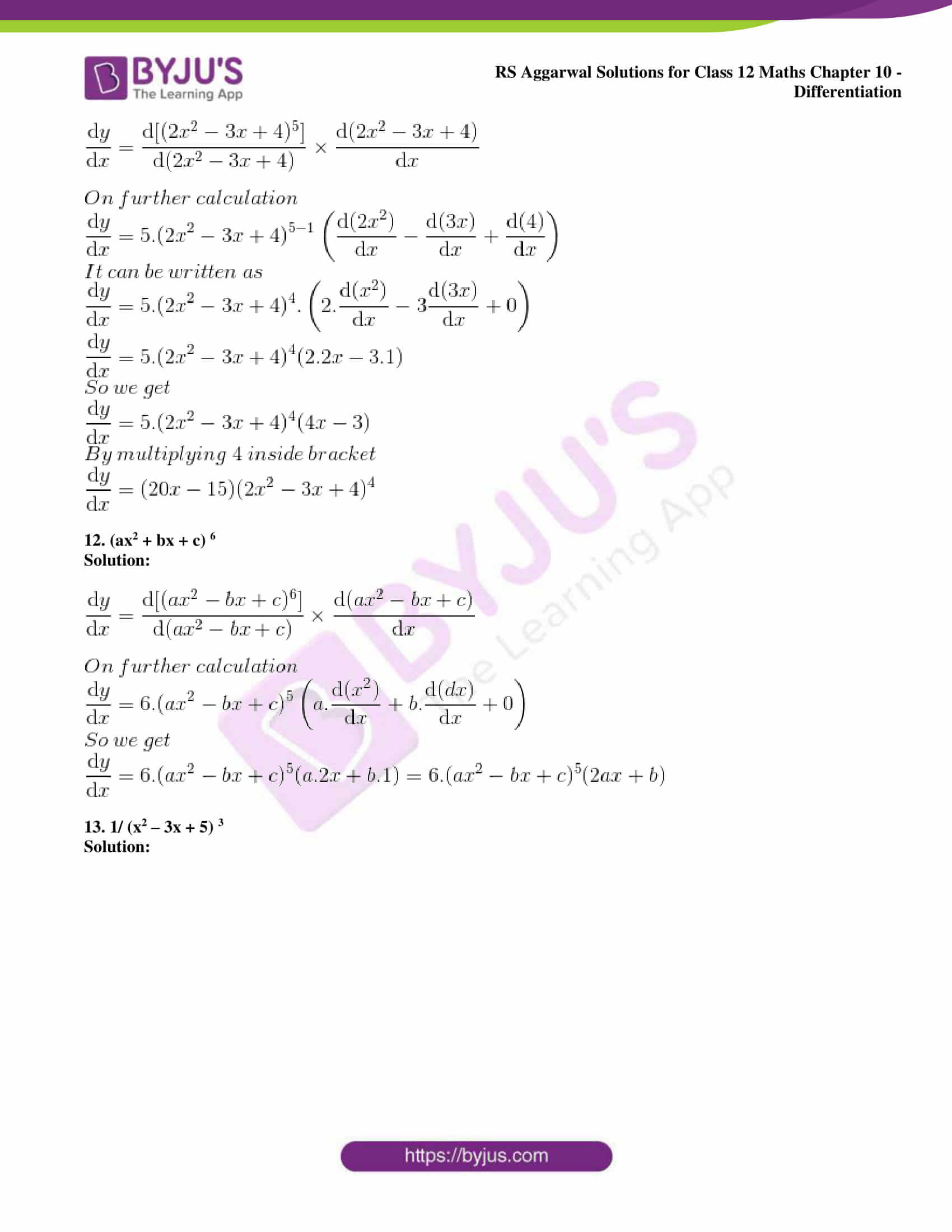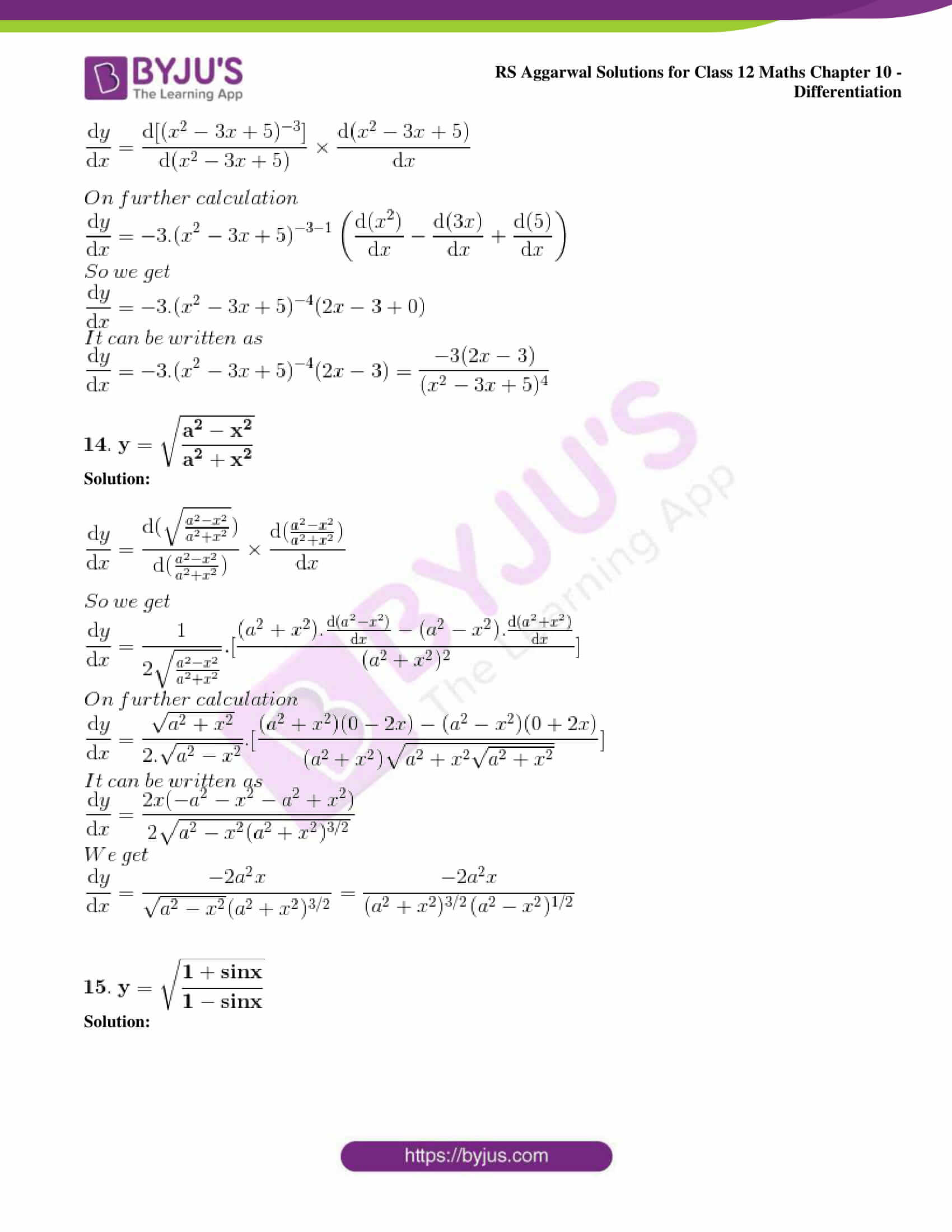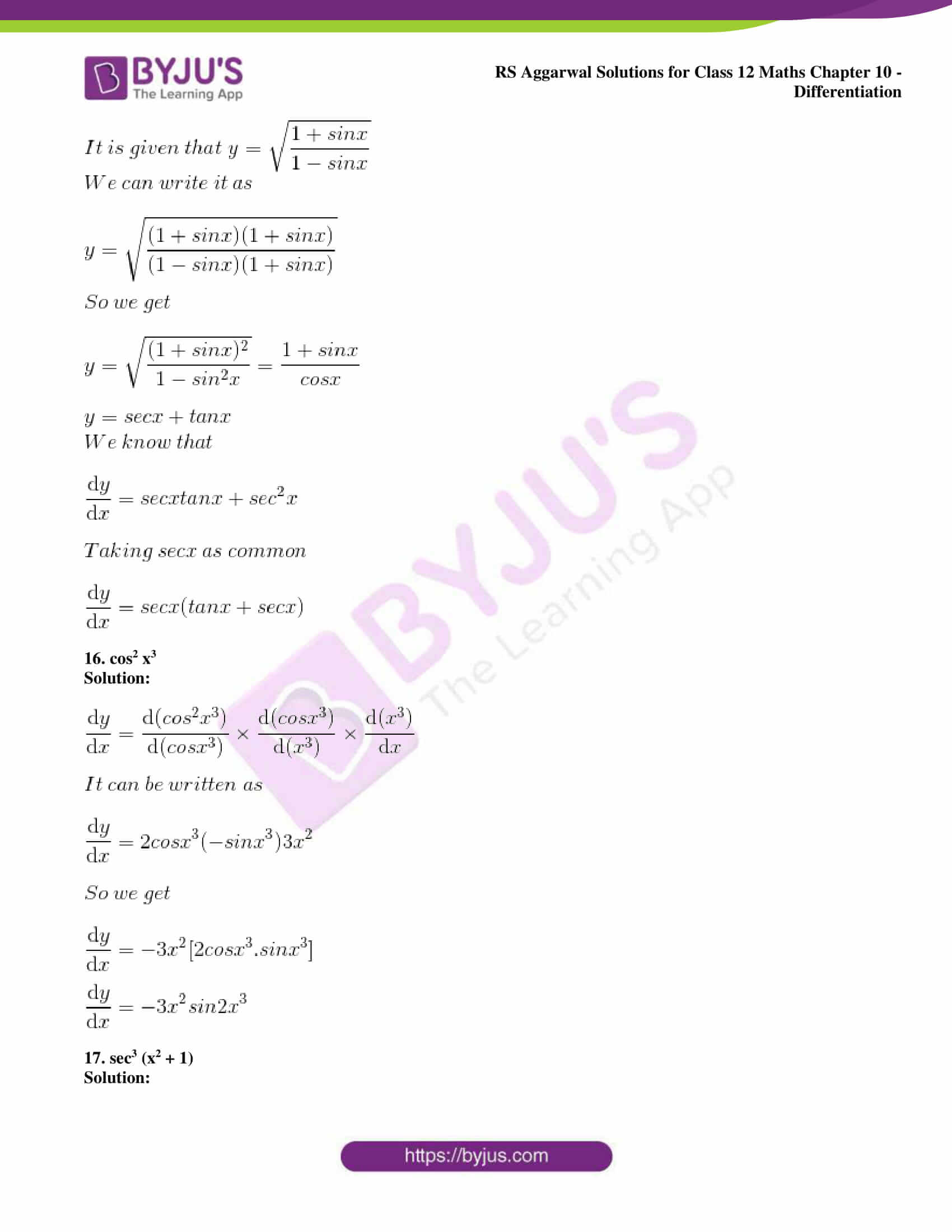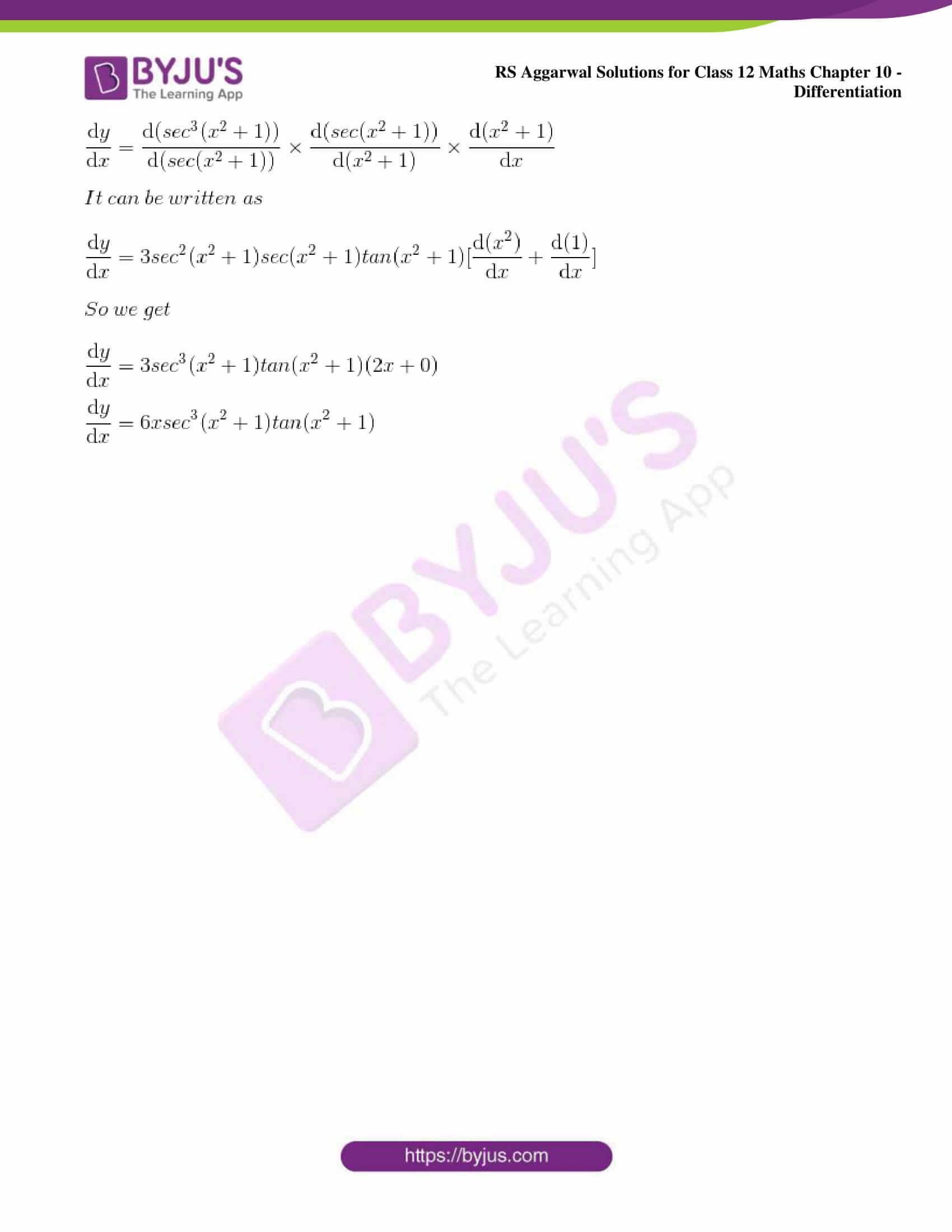### Access other exercise solutions of Class 12 Maths Chapter 10: Differentiation

Exercise 10B Solutions

Exercise 10C Solutions

Exercise 10D Solutions

Exercise 10E Solutions

Exercise 10F Solutions

Exercise 10G Solutions

Exercise 10H Solutions

Exercise 10I Solutions

Exercise 10J Solutions

### Access RS Aggarwal Solutions for Class 12 Maths Chapter 10: Differentiation Exercise 10A

Differentiate each of the following w.r.t. x:

1. sin 4x

Solution:

By differentiating both sides w.r.t. x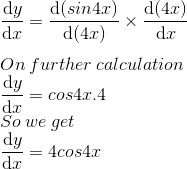2. cos 5x

Solution: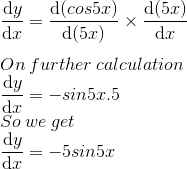3. tan 3x

Solution: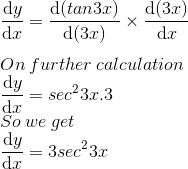4. cos x3

Solution: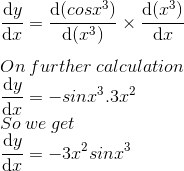5. cot2 x

Solution: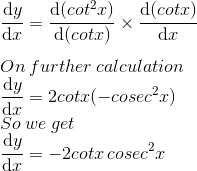6. tan3 x

Solution: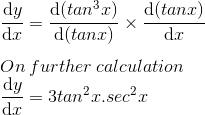7. cot √x

Solution: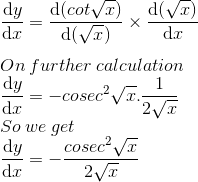8. √tan x

Solution: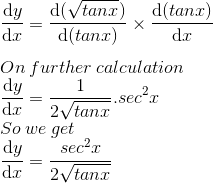9. (5 + 7x) 6

Solution: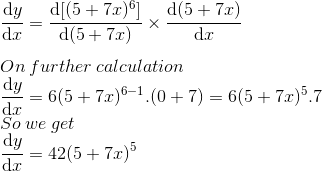10. (3 – 4x) 5

Solution: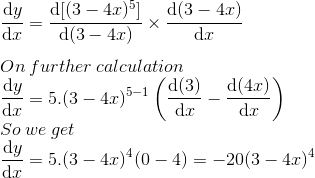11. (2x2 – 3x + 4) 5

Solution: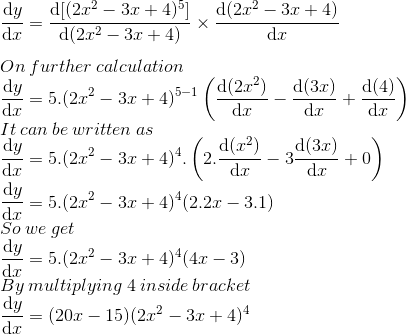12. (ax2 + bx + c) 6

Solution: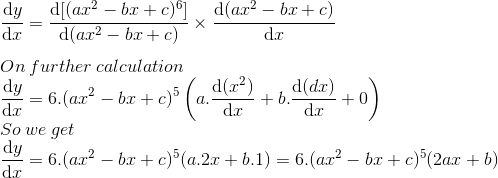13. 1/ (x2 – 3x + 5) 3

Solution: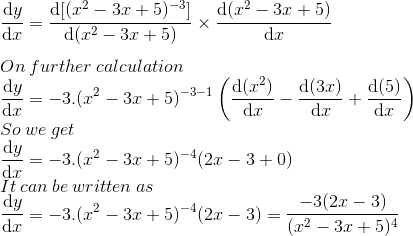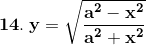Solution: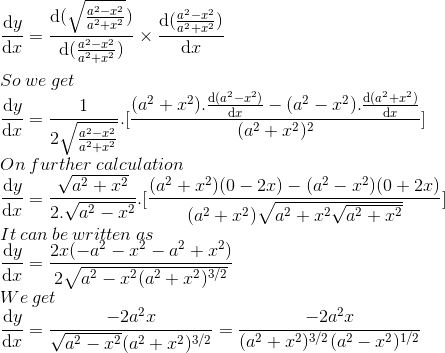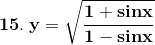Solution: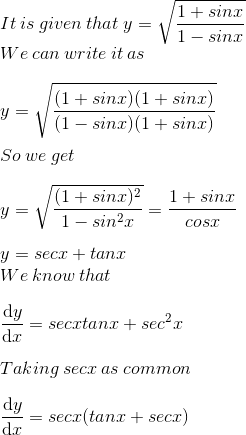16. cos2 x3

Solution: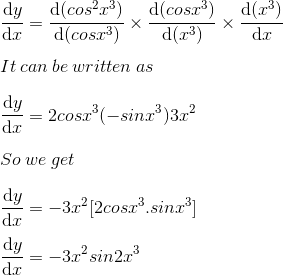17. sec3 (x2 + 1)

Solution: